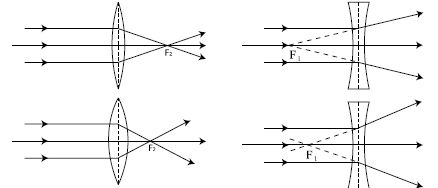# What is Power of Lens

Suppose there are two convex lenses. The focal length of the fast one is greater and the focal length of the second one is smaller.Now if a beam of parallel rays of light parallel to the principal axis incidents on the lens then after refraction the rays will meet at the principal focus. In the case of the second lens, that focal point will not be as far as the first one, rather it will be nearer. The power of a convex lens is the ability to convert a parallel beam of light into a convergent beam. Therefore it can be said that the power of the first lens is less than that of the second one. The power of a lens is greater if its focal length is less and the power will be less if the focal length is greater.

In figure2, the refraction of parallel rays in concave lens is shown. In the case of concave lens the ability to convert a parallel beam of light into a divergent beam is called its power. The more the concave lens can diverge a beam of parallel rays the more is its power. That is the shorter the focal length of a lens, the greater is its power. In general, we can say that the capacity of a lens to convert a parallel beam of light into a convergent beam or into a divergent beam is called the power of the lens.

There is a relation between the power P and focal length £ That is, P = 1/f

The power of lens of focal length one meter is called one diapter (d). The power of spectacle that the eye specialists recommend is written in diapter unit.